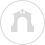# Math: Calculus Information

### Students who wish to register for Precalculus or Calculus I are required to take the ACCUPLACER Calculus Placement Test prior to course registration.

The Calculus Placement test assesses from intermediate algebra through pre-calculus. This includes:

• Algebraic operations includes simplifying rational algebraic expressions, factoring, expanding polynomials, and manipulating roots and exponents.
• Solutions of equations and inequalities includes the solution of linear and quadratic equations and inequalities, equation systems and other algebraic equations.
• Coordinate geometry includes plane geometry, the coordinate plane, straight lines, conics, sets of points in the plane, and graphs of algebraic functions.
• Applications and other algebra topics ask about complex numbers, series and sequences, determinants, permutations and combinations, fractions, and word problems.
• The last category, functions and trigonometry, presents questions about polynomials, algebraic, exponential, logarithmic and trigonometric functions.

Students who score 4 or 5 on AP Calculus AB receive credit for Calculus I and are not required to take the Calculus Placement Test.

Students who score 4 or 5 on AP Calculus BC receive credit for Calculus I and II and are not required to take the Calculus Placement Test.

Students who score 3 on AP Calculus AB or AP Calculus BC receive no credit but may register for MATH 121 Calculus I and are not required to take the Calculus Placement Test.